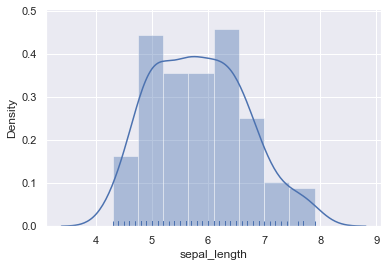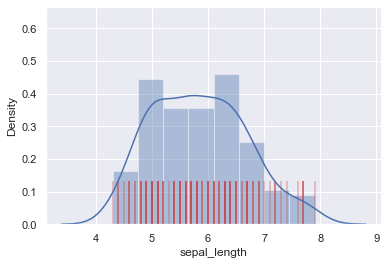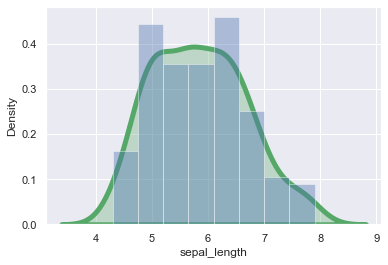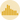# Control rug and density on a Seaborn histogram

By default, the hisplot function of Seaborn plots an histogram without a density curve (see graph #20). You can easily add the latter by setting the kde argument to True. You can also control the presence of rugs using rug='True'. You can customize rug and density as presented below.

## Histogram + rug + kernel density curve

``````# libraries & dataset
import seaborn as sns
import matplotlib.pyplot as plt
# set a grey background (use sns.set_theme() if seaborn version 0.11.0 or above)
sns.set(style="darkgrid")

sns.distplot(df["sepal_length"], kde=True, rug=True)
# in the next version of the distplot function, one would have to write:
# sns.distplot(data=df, x="sepal_length", kde=True, rug=True) # note that 'kind' is 'hist' by default
plt.show()``````## Customizing rugs

``````# libraries & dataset
import seaborn as sns
import matplotlib.pyplot as plt
# set a grey background (use sns.set_theme() if seaborn version 0.11.0 or above)
sns.set(style="darkgrid")

sns.distplot(df["sepal_length"],
kde=True,
rug=True,
rug_kws={"color": "r", "alpha": 0.3, "linewidth": 2, "height":0.2})
# in the next version of the distplot function, one would have to write:
# sns.distplot(data=df, x="sepal_length", kde=True, rug=True, rug_kws={"color": "r", "alpha":0.3, "linewidth": 2, "height":0.2 })
plt.show()``````## Customizing the density curve

``````# libraries & dataset
import seaborn as sns
import matplotlib.pyplot as plt
# set a grey background (use sns.set_theme() if seaborn version 0.11.0 or above)
sns.set(style="darkgrid")

sns.distplot(df["sepal_length"],
kde=True,
kde_kws={"color": "g", "alpha": 0.3, "linewidth": 5, "shade": True})
# in the next version of the distplot function, one would have to write:
# sns.distplot(data=df, x="sepal_length", kde=True, kde_kws={"color": "g", "alpha": 0.3, "linewidth": 5, "shade": True})
plt.show()``````## Contact & Edit

👋 This document is a work by Yan Holtz. Any feedback is highly encouraged. You can fill an issue on Github, drop me a message onTwitter, or send an email pasting `yan.holtz.data` with `gmail.com`.

This page is just a jupyter notebook, you can edit it here. Please help me making this website better 🙏!